# Binomial HeapAs we described in the previous post, leftist tree is a binary tree based functional heap. It manipulates the tree structure so that the left branches are always the longest and operations follow the right branches only. It is a clever and simple data structure that fulfills the purpose of heap.

In this post, we present another functional heap called Binomial Heap (). Instead of being a single tree strucutre, it is a list of binomial trees and it provides better performance than leftist tree on insert.

However, the reason I would like to talk about it here is not because of its efficiency. The fascination of binomial heap is that if there are N elements inside it, then it will have a determined shape, no matter how it ended up with the N elements. Personally I find this pretty cool. This is not common in tree related data structures. For example, we can not predict the shape of a leftist tree with N elements and the form of a binary search tree can be arbitrary even if it is somehow balanced.

Let's have a close look at it.

# Binomial Tree

Binomial tree is the essential element of binomial heap. Its special structure is why binomial heap exists. Understanding binomial tree makes it easier to understand binomial heap.

Binomial tree's definition does not involve the values associated with the nodes, but just the structure:

1. It has a rank r and r is a natural number.
2. Its form is a root node with a list of binomial trees, whose ranks are strictly r-1, r-2, ..., 0.
3. A binomial tree with rank 0 has only one root, with an empty list.

Let's try producing some examples.

From point 3, we know the base case: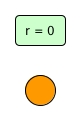Now, how about rank 1? It should be a root node with a sub binomial tree with rank 1 - 1 = 0: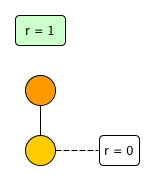Let's continue for rank 2, which should have rank 1 and rank 0: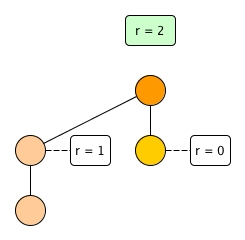Finally rank 3, can you draw it?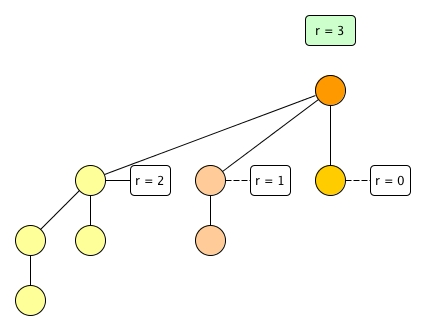## $2^r$ nodes

If we pull up the left most child of the root, we can see: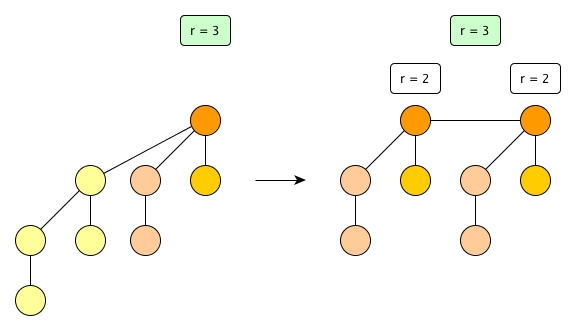This means a binomial tree with rank r can be seen as two binomial trees with the same rank r-1. Furthermore, because that two, and rank 0 has one node, then in term of the number of nodes, for a binomial tree with rank r, it must have $2^r$ nodes, no more, no less.

For example, rank 0 has 1 node. Rank 1 is 2 rank 0, so rank 1 has $2 * 1 = 2$ nodes, right? Rank 2 then has $2 * 2 = 4$ nodes, and so on so forth.

Note that $2^r = 1 + 2^r-1 + 2^r-2 + ... + 2^0$ and we can see that a rank r tree's structure fits exactly to this equation (the 1 is the root and the rest is the children list).

## Two r-1 is the way to be r

The definition tells us that a rank r tree is a root plus a list of trees of rank r-1, r-2, ..., and 0. So if we have a binomial tree with an arbitrary rank, can we just insert it to another target tree to form a rank r tree?

For example, suppose we have a rank 1 tree, can we insert it to the target tree below for a rank 3 tree?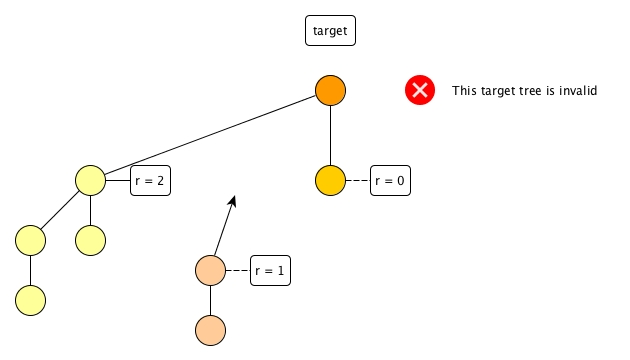Unfortunately we cannot, because the target tree won't be able to exist in the first place and it is not a valid binomial tree, is it?

Thus in order to have a rank r tree, we must have two r-1 trees and link them together. When linking, we need to decide which tree is the new root, depending on the context. For the purpose of building a min heap later, we assume we always let the root with the smaller key be the root of the new tree.

## code

Defining a binomial tree type is easy:

(* Node of key * child_list * rank *)
type 'a binomial_t = Node of 'a * 'a binomial_t list * int


Also we can have a function for a singleton tree with rank 0:

let singleton_tree k = Node (k, [], 0)


Then we must have link function which promote two trees with same ranks to a higher rank tree.

let link ((Node (k1, c1, r1)) as t1) ((Node (k2, c2, r2)) as t2) =
if r1 <> r2 then failwith "Cannot link two binomial trees with different ranks"
else if k1 < k2 then Node (k1, t2::c1, r1+1)
else Node (k2, t1::c2, r2+1)


One possibly interesting problem can be:

Given a list of $2^r$ elements, how to construct a binomial tree with rank r?

We can borrow the idea of merging from bottom to top for this problem.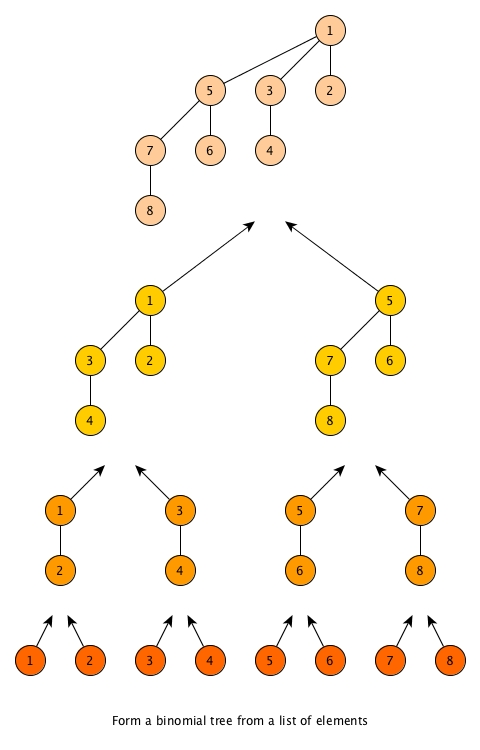let link_pair l =
let rec aux acc = function
| [] -> acc
| _::[] -> failwith "the number of elements must be 2^r"
| t1::t2::tl -> aux (link t1 t2 :: acc) tl
in
aux [] l

let to_binomial_tree l =
let singletons = List.map singleton_tree l in
let rec aux = function
| [] -> failwith "Empty list"
| t::[] -> t
| l -> aux (link_pair l)
in
aux singletons


## Binomial coefficient

If we split a binomial tree into levels and pay attention to the number of nodes on each level, we can see: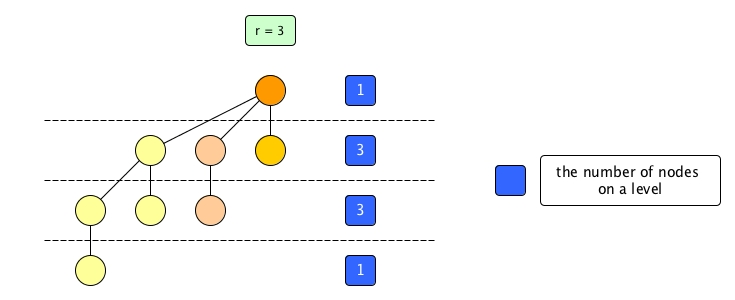So from top to bottom, the numbers of nodes on levels are 1, 3, 3 and 1. It happens to be the coefficients of $(x+y)^3$ .

Let's try rank 4: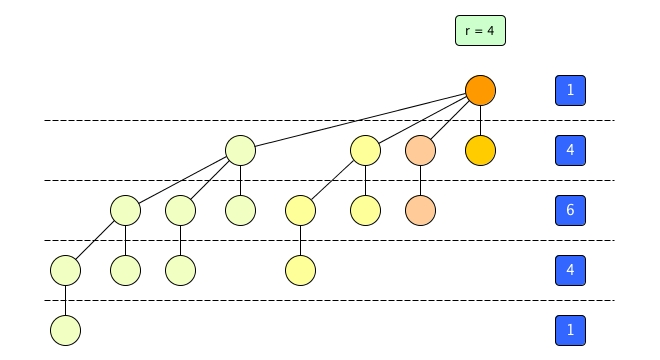They are 1, 4, 6, 4 and 1, which are the coefficients of $(x+y)^4$ .

The number of nodes on level k ( 0 <= k <= r) matches ${r}\choose{k}$, which in turn matches the kth binomial coefficient of $(x+y)^r$. This is how the name binomial tree came from.

# Binomial Heap

A binomial heap is essentially a list of binomial trees with distinct ranks. It has two characteristics:

1. If a binomial heap has n nodes, then its shape is determined, no matter what operations have been gone through it.
2. If a binomial heap has n nodes, then the number of trees inside is O(logn).

The reason for the above points is explained as follows.

As we already knew, a binomial tree with rank r has $2^r$ nodes. If we move to the context of binary presentation of numbers, then a rank r tree stands for the case where there is a list of bits with only the rth slot turned on.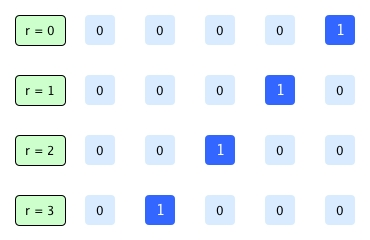Thus, for n number of nodes, it can be expressed as a list of binomial trees with distinct ranks, because the number n is actually a list of bits with various slots being 1. For example, suppose we have 5 nodes (ignoring their values for now), mapping to a list of binomial trees, we will have: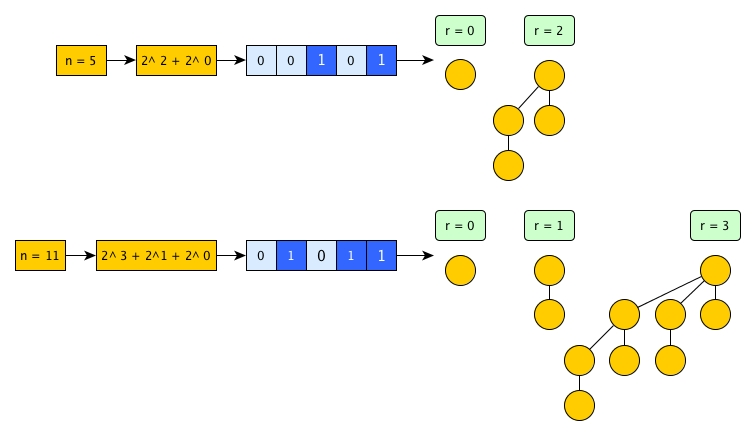This is where binomial heap comes from.

1. Since a number n has determined binary presentation, a binomial heap also has fixed shape as long as it has n nodes.
2. In addition, because n has O(logn) effective bits, a binomial heap has O(logn) binomial trees.
3. If we keep each binomial tree having the min as the root, then for a binomial heap, the overall minimum elements is on of those roots.

Let's now implement it.

## Type and singleton

It is easy.

type 'a binomial_heap_t = 'a binomial_t list


## insert

When we insert a key k, we just create a singleton binomial tree and try to insert the tree to the heap list. The rule is like this:

1. If the heap doesn't have a rank 0 tree, then directly insert the new singleton tree (with rank 0) to the head of the list.
2. If the heap has a rank 0 tree, then the two rank 0 tree need to be linked and promoted to a new rank 1 tree. And we have to continue to try to insert the rank 1 tree with the rest of the list that potentiall starts with a existing rank 1 tree.
3. If there is already a rank 1 tree, then link and promot to rank 2... so on so forth, until the newly promoted tree has a slot to fit in.

Here are two examples: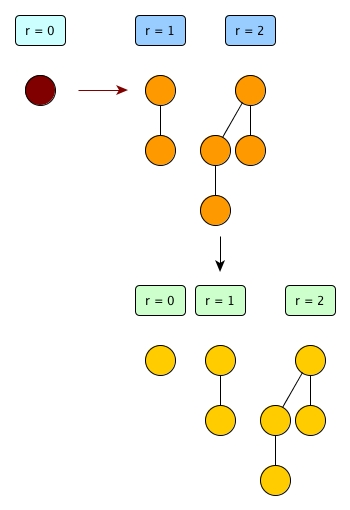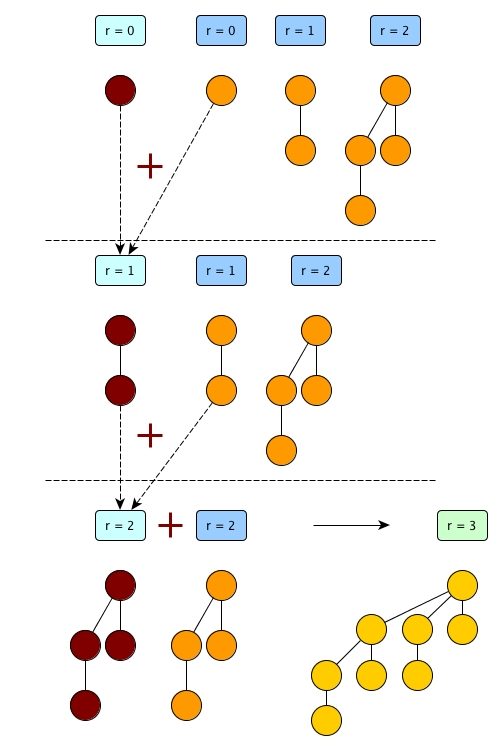The insert operation is actually the addition between 1 and n in binary presentation, in a revered order.

let insert k h =
let rec aux acc (Node (_, _, r1) as bt1) = function
| [] -> List.rev (bt1::acc)
| (Node (_, _, r2) as bt2)::tl ->
if r1 = r2 then aux acc (link bt1 bt2) tl
else if r1 < r2 then List.rev_append acc (bt1::bt2::tl)
else aux (bt2::acc) bt1 tl
in
aux [] (singleton_tree k) h


If the heap is full as having a consecutive series of ranks of trees starting from rank 0, we need O(logn) operations to finish the insert. However, once it is done, most of the lower rank slots are empty (like shown in the above figure). And for later new insert, it won't need O(logn) any more. Thus, The time complexity of insert seems to be O(logn), but actually amortised O(1).

Note the above insert description is just for demonstration purpose. Like in Leftist tree, merge is the most important operation for binomial heap and insert is just a simpler merge.

## merge

The merge is like this:

1. Get the two heads (bt1 and bt2) out of two heaps (h1 and h2).
2. If rank bt1 < rank bt2, then bt1 leaves first and continue to merge the rest of h1 and h2.
3. If rank bt1 > rank bt2, then bt2 leaves first and continue to merge h1 and the rest of h2.
4. If rank bt1 = rank bt2, then link bt1 bt2, add the new tree to the rest of h1 and merge the new h1 and the rest of h2.

I will skip the digram and directly present the code here:

let rec merge h1 h2 =
match h1, h2 with
| h, [] | [], h -> h
| (Node (_, _, r1) as bt1)::tl1, (Node (_, _, r2) as bt2)::tl2 ->
if r1 < r2 then bt1::merge tl1 h2
else if r1 > r2 then bt2::merge h1 tl2
else merge (link bt1 bt2::tl1) tl2

(* a better and simpler version of insert *)
let insert' k h = merge [singleton_tree k] h


The time complexity is O(logn).

## get_min

We just need to scan all roots and get the min key.

let get_min = function
| [] -> failwith "Empty heap"
| Node (k1, _, _)::tl ->
List.fold_left (fun acc (Node (k, _, _)) -> min acc k) k1 tl


For achieve O(1), we can attach a minimum property to the heap's type. It will always record the min and can be returned immediately if requested. However, we need to update this property when insert, merge and delete_min. Like every other book does, this modification is left to the readers as an exercise.

## delete_min

delete_min appears as a little bit troublesome but actually very neat.

1. We need to locate the binomial tree with min.
2. Then we need to merge the trees on its left and the trees on its right to get a new list.
3. It is not done yet as we need to deal with the min binomial tree.
4. We are lucky that a binomial tree's child list is a heap indeed. So we just need to merge the child list with the new list from point 2.
let key (Node (k, _, _)) = k
let child_list (Node (_, c, _)) = c

let split_by_min h =
let rec aux pre (a, m, b) = function
| [] -> List.rev a, m, b
| x::tl ->
if key x < key m then aux (x::pre) (pre, x, tl) tl
else aux (x::pre) (a, m, b) tl
in
match h with
| [] -> failwith "Empty heap"
| bt::tl -> aux [bt] ([], bt, []) tl

let delete_min h =
let a, m, b = split_by_min h in
merge (merge a b) (child_list m |> List.rev)


# Binomial Heap vs Leftist Tree

|               | get_min                                 | insert         | merge   | delete_min |
|---------------|-----------------------------------------|----------------|---------|------------|
| Leftist tree  | O(1)                                    | O(logn)        | O(logn) | O(logn)    |
| Binomial heap | O(logn), but can be improved to be O(1) | Amortised O(1) | O(logn) | O(logn)    |



 Binomial Heap is also introduced in Purely Functional Data Structures.